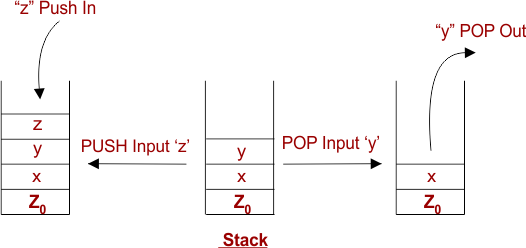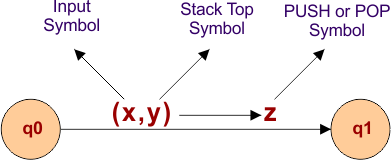Select Page

# Pushdown Automata Introduction

A pushdown automaton (PDA) is a way to implement a context-free grammar (CFG) in a similar way we design Finite Automata (FA) for a regular grammar (RG). A DFA has limited memory, but a PDA can hold unlimited memory.

Basically a Pushdown Automaton is

“Finite Automata Machine” + “a stack”, where

• FA represents a finite memory for finite number of states
• And stack is an extra memory with infinite size to PUSH and POP values.

## Operation of Pushdown Automata

There are two basic Operations in Pushdown Automata.

1. PUSH: It means insertion a new input symbol into the stack.

2. POP: It means removal a input symbol from the stack.Note: PUSH and POP operations are always performed on the top of stack

## Components of Pushdown Automata

A pushdown automaton has 3 basic components

• Input tape: It contains input string
• Control unit: It takes an input and forwards it to stack for PUSH or POP.
• Stack: It is memory of infinite size.

The following diagram explains the above components.Note: A PDA may or may not PUSH or POP an input symbol in every transition. But PDA has to read the top of the stack in every transition.

## 7-Tuples of Pushdown Automata

Pushdown Automata (PDA) can be formally described by 7-tuples (Q, ∑, Γ, δ, q0, Z0, F)

• Qis the finite number of states
• is input alphabet which are PUSH or POP from Stack.
• Γis stack symbols.
• δis the transition function: Q × (∑ ∪ {ε}) x Γ  à Q × Γ *   // where Q is any state ∑ is input symbol, ε is epsilon, Γ is top of the stack value and  Γ * represents all symbols existing in stack.
• q0is the start state (q0 ∈ Q)
• Z0is the default value of stack. It is used to represent the Null value of stack. We can say Z0 is an epsilon.
• Fis a set of Final states (F ∈ Q). There may or may not exist Final states in PDA.

## Transition Function in PDA

Transition function depends on current state and three symbols

• Input symbol
• Stack Top Symbol
• Push Symbol

The Value of any symbols (Input or stack or push) can be epsilon (∈).

Let us see the following diagram which shows a transition in a PDA from a state q0 to state q1, labeled as x,y → zThis means at state q0, if we consume an input string ‘x’ and top symbol of the stack is ‘y’, then we pop ‘y’ and push ‘z’ on top of the stack and move to next state q1.

Help Other’s By Sharing…